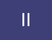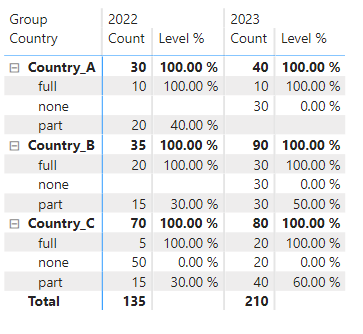cancel
Showing results for
Did you mean:Regular Visitor

## How to calculate total Sum in Matrix visual with complex calculation?

I am stuck with a problem for some time now, and hope to get some help here.
My data contains these for example:"Count" is the number of available positions in our case, "level" the percentage of how much of this position is allready financed. Every Country can have different amounts of positions with different levels in different years.

What I finally want to show is drawn in this dummy (red numbers are calculated by hand an inserted in the screenshot ;-).
The totals should show the level on either country or total perspective, taking in count the part the level has on a row base compared to the sum of count for the total country (or overall on the bottom).I manage to get the part for every type (full, part, none), as shown above as "Part of Country". But I have no idea how to calculate the percentage on a higher level, which is the sum of them. Even more complicated (for me): Getting the value for the overall perspective ("Gesamt", german).What can I do to get results like in the dummy? The pbix for this demo can be found here.

1 ACCEPTED SOLUTIONHelper I

Hi @OliTFD check out my blog post on this (I had a similar case where I wanted to sum quantities per productid , I am pretty sure you will find it useful). It is based on the SUM() OVER PARTITION BY of SQL , I think you can also use the same logic for your COUNT https://www.iipowerblog.com/post/sum-over-partition-by-in-powerbi

6 REPLIES 6Regular Visitor

@IIPowerBlog  At last your idea gave me the solution! I need to drop the measures, and do the calculations in columns, to finally sum them.

First step: Get the count for the country
Total Count per Country COL = CALCULATE(SUM(Facts[Count]),ALLEXCEPT(Facts,Facts[Group],Facts[Country]))

Second: Calculate the count compared to Country

Count compared to Country COL = DIVIDE(Facts[Count],Facts[Total Count per Country COL])

Third: Multiply with level
Part of Country COL = Facts[Count compared to Country COL]*Facts[Level]
Finally sum this for the country level, same method for the group level. And then adjust the visible column in the matrix to
Level % =
Var Typelevel = Sum(Facts[Level])
Var Countrylevel = Sum(Facts[Part of Country COL])
Var Grouplevel = Sum(Facts[Part of Group COL])
Return

If(
ISINSCOPE(Facts[type]),Typelevel,
If(ISINSCOPE(Facts[Country]),Countrylevel,Grouplevel))

This gives the following view:Problem solved (and found a typo in my dummy ;-))Regular Visitor

@IIPowerBlog thanks, but this does not seem to work. The Calculate(Sum(... needs a column... but I need to sum values of a measure, which depends on another measure.
As far as I see your solution does more or less the same as my "Total Count per Country)-measure from step 1.
Or do I get things wrong?Helper I

Hi @OliTFD check out my blog post on this (I had a similar case where I wanted to sum quantities per productid , I am pretty sure you will find it useful). It is based on the SUM() OVER PARTITION BY of SQL , I think you can also use the same logic for your COUNT https://www.iipowerblog.com/post/sum-over-partition-by-in-powerbiRegular Visitor

@amitchandak , AverageX is not the solution, the calculation is a little bit more complex, see below
@v-rzhou-msft Thanks, this part is not the main problem, but thanks for the advise. To get the problem clearer, I try to explain the calculation with the image below:It is a kind of three-steps-calculation:
- compare the count to the total for country
- multiply this with the level
- sum up this results for the country

The red part is where I am lost ;-). This needs to be the _HAND_CALCULATE-Part.
For the overall total ("Gesamt") it will be more or less the same, just changing step 1 to "compare to total for group".Community Support

Hi @OliTFD ,

According to your statement, I think your want to get subtoatl in country level by another calculate logic. However, I am still confused about your calculate logic for red part in your screenshot. Here I suggest you to try ISINSCOPE() function to achieve your goal. Here I use 1 in hand calculate part.

``````Level % =
VAR _HAND_CALCULATE = 1
RETURN
IF (
ISINSCOPE ( Facts[Type] ),
SUM ( Facts[Level] ),
IF ( ISINSCOPE ( Facts[Country] ), _HAND_CALCULATE )
)``````

Result is as below.Best Regards,
Rico Zhou

If this post helps, then please consider Accept it as the solution to help the other members find it more quickly.Super User

@OliTFD , for the red one, try a measure like

AverageX(Table, [Count]*[Level])Announcements#### Exclusive opportunity for Women!

Join us for a free, hands-on Microsoft workshop led by women trainers for women where you will learn how to build a Dashboard in a Day!#### Power Platform Conference-Power BI and Fabric Sessions

Join us Oct 1 - 6 in Las Vegas for the Microsoft Power Platform Conference.Top Solution Authors
Top Kudoed Authors
Users online (1,988)